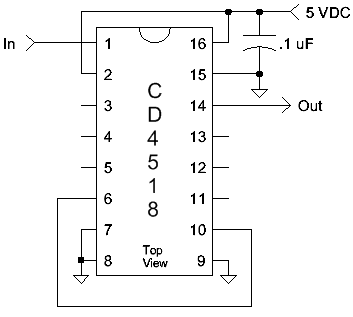###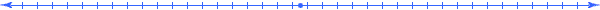## CD4069 Atomic Frequency Standard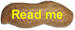Like the Two-Transistor Atomic Frequency Standard, the CD4069 Atomic Frequency Standard picks up AC "hum" from the surrounding environment to produce an accurate frequency reference. This simple circuit generates 10 Hz and 20 Hz from 60 Hz power grids.

A short antenna picks up 60 Hz from the building wiring and applies the signal to a linearized gate, acting as a sensitive amplifier (pins 1 and 2). The 0.1 uF capacitor to ground at the junction of the two, 10 megohm resistors prevents negative feedback from lowering the input resistance, so the amplifier is quite sensitive. The output of the amplifier is applied to the second gate where it is squared up (pins 3 and 4). A 60 Hz square wave should be observed on the 10 k resistor connected to pin 4. Little "glitches" don't matter too much as they will be removed by the following injection-locked oscillators. The next two gates form an oscillator operating at 20 Hz and this oscillation is strongly injection-locked to the 60 Hz input. The 20 Hz output is divided by 2 in the next section in a similar manner.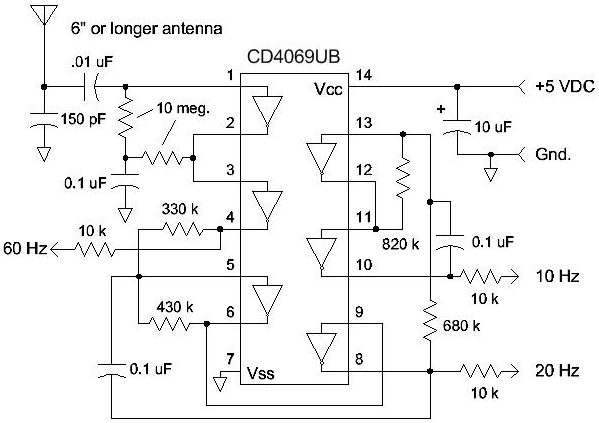If your power system uses 50 Hz, change the 330 k and 430 k to 680 k and 820 k. That should divide by 5 and give 10 Hz at pin 8. Use a 0.22 uF for that last 0.1 to get 5 Hz out of the last stage. A little tweaking of the values may be necessary.

The standard values shown should work fine, but the experimenter may fine-tune the circuit by connecting a generator to the antenna and ground and finding a value for the 330 k or 430 k resistor (either is fine) that gives a symmetrical divide-by-3 around 60 Hz. Then repeat the operation to find a value for the 680 k or 820 k resistor that gives the final divide-by-6 with the widest deviation from 60 Hz. Doing this little tweak will improve the weak signal performance and reduce the likelihood that the circuit will start dividing by the wrong number at some future date.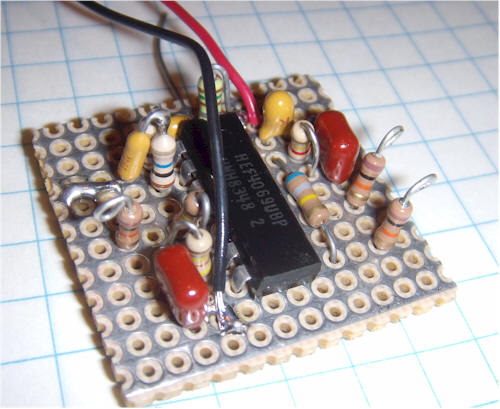This little board will drive an additional divide-by 100 to get 10 second pulses for a data taker. For no particular reason, I potted the board in a plastic box, using potting wax. There's a bolt sticking out the back for mounting.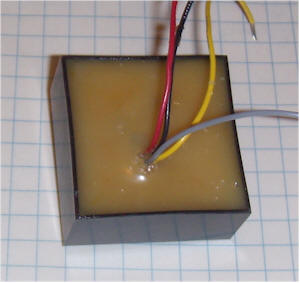Here's a simple divide-by-100 circuit that may be added to any of the outputs. Adding this divider to the 10 Hz output gives a pulse every 10 seconds.